Plot Dataset Level Model Profile Explanations

# S3 method for model_profile
plot(x, ..., geom = "aggregates")

## Arguments

x a variable profile explanation, created with the model_profile function other parameters either "aggregates", "profiles", "points" determines which will be plotted

## Value

An object of the class ggplot.

### aggregates

• color a character. Either name of a color, or hex code for a color, or _label_ if models shall be colored, or _ids_ if instances shall be colored

• size a numeric. Size of lines to be plotted

• alpha a numeric between 0 and 1. Opacity of lines

• facet_ncol number of columns for the facet_wrap

• variables if not NULL then only variables will be presented

• title a character. Partial and accumulated dependence explainers have deafult value.

• subtitle a character. If NULL value will be dependent on model usage.

## Examples

titanic_glm_model <- glm(survived~., data = titanic_imputed, family = "binomial")
explainer_glm <- explain(titanic_glm_model, data = titanic_imputed)
#> Preparation of a new explainer is initiated
#>   -> model label       :  lm  (  default  )
#>   -> data              :  2207  rows  8  cols
#>   -> target variable   :  not specified! (  WARNING  )
#>   -> predict function  :  yhat.glm  will be used (  default  )
#>   -> predicted values  :  No value for predict function target column. (  default  )
#>   -> model_info        :  package stats , ver. 4.1.1 , task classification (  default  )
#>   -> model_info        :  Model info detected classification task but 'y' is a NULL .  (  WARNING  )
#>   -> model_info        :  By deafult classification tasks supports only numercical 'y' parameter.
#>   -> model_info        :  Consider changing to numerical vector with 0 and 1 values.
#>   -> model_info        :  Otherwise I will not be able to calculate residuals or loss function.
#>   -> predicted values  :  numerical, min =  0.008128381 , mean =  0.3221568 , max =  0.9731431
#>   -> residual function :  difference between y and yhat (  default  )
#>   A new explainer has been created!  expl_glm <- model_profile(explainer_glm, "fare")
plot(expl_glm)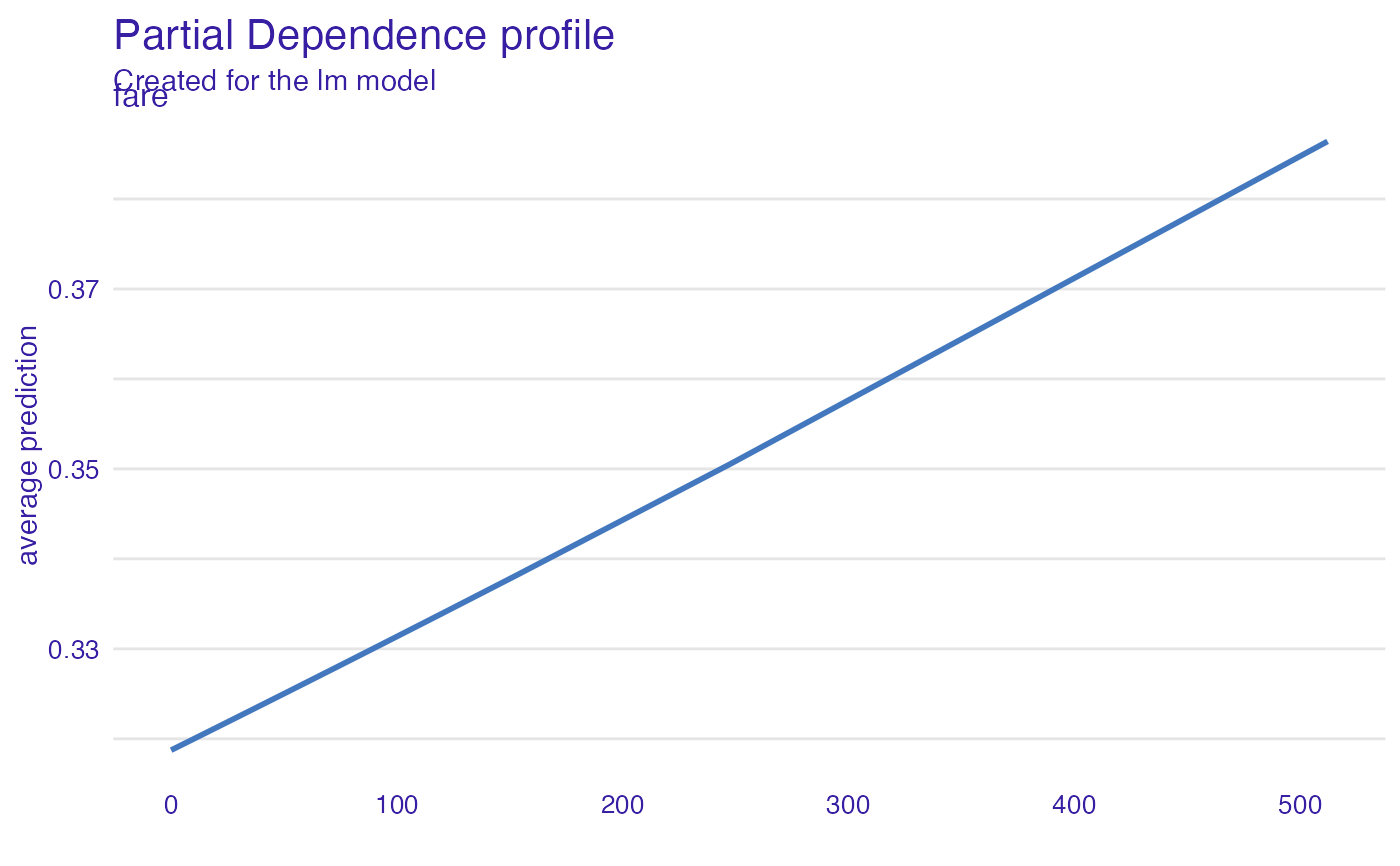# \donttest{
library("ranger")
titanic_ranger_model <- ranger(survived~., data = titanic_imputed, num.trees = 50,
probability = TRUE)
explainer_ranger  <- explain(titanic_ranger_model, data = titanic_imputed)
#> Preparation of a new explainer is initiated
#>   -> model label       :  ranger  (  default  )
#>   -> data              :  2207  rows  8  cols
#>   -> target variable   :  not specified! (  WARNING  )
#>   -> predict function  :  yhat.ranger  will be used (  default  )
#>   -> predicted values  :  No value for predict function target column. (  default  )
#>   -> model_info        :  package ranger , ver. 0.13.1 , task classification (  default  )
#>   -> model_info        :  Model info detected classification task but 'y' is a NULL .  (  WARNING  )
#>   -> model_info        :  By deafult classification tasks supports only numercical 'y' parameter.
#>   -> model_info        :  Consider changing to numerical vector with 0 and 1 values.
#>   -> model_info        :  Otherwise I will not be able to calculate residuals or loss function.
#>   -> predicted values  :  numerical, min =  0.007755556 , mean =  0.3218902 , max =  0.9938384
#>   -> residual function :  difference between y and yhat (  default  )
#>   A new explainer has been created!  expl_ranger <- model_profile(explainer_ranger)
plot(expl_ranger)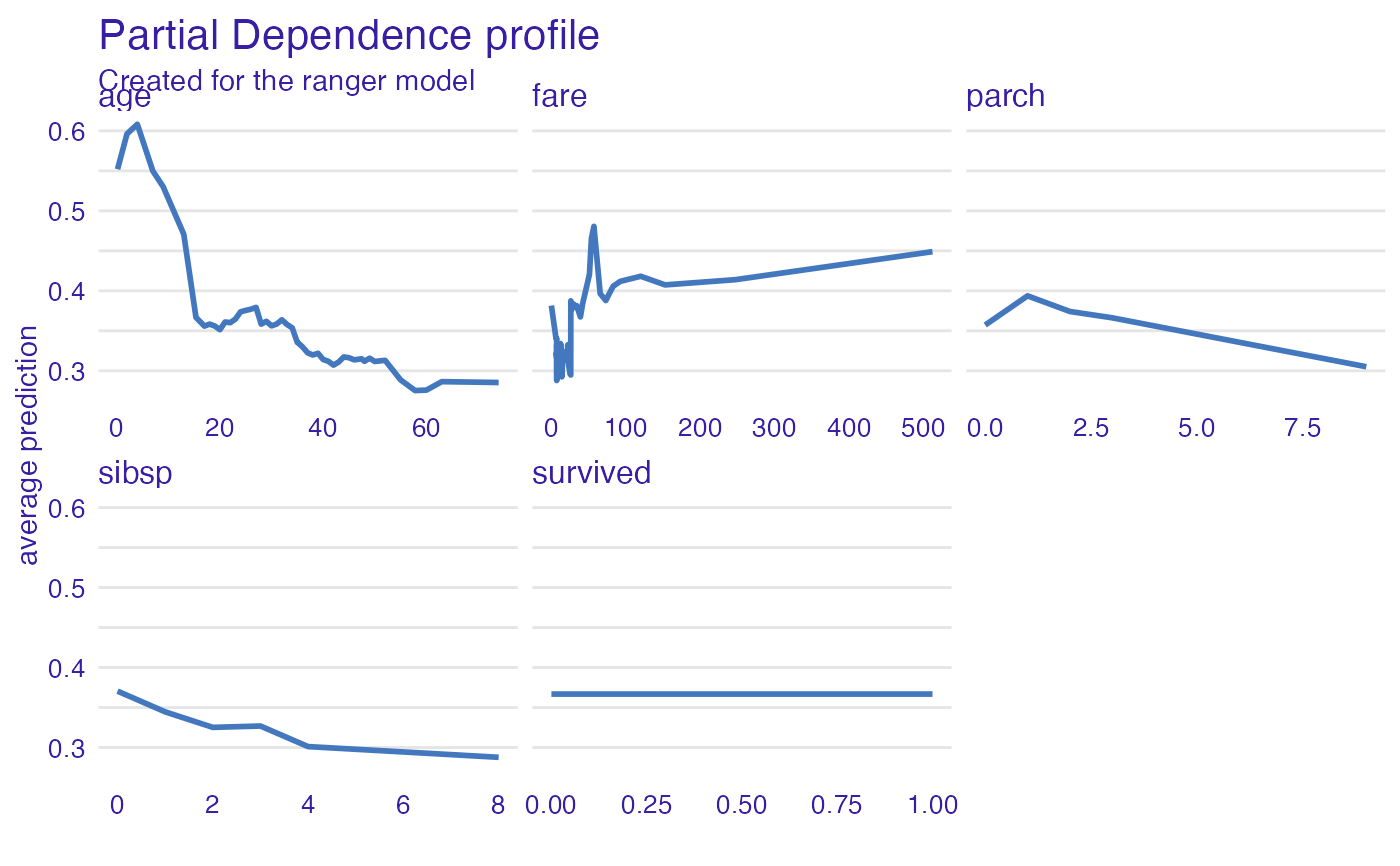plot(expl_ranger, geom = "aggregates")vp_ra <- model_profile(explainer_ranger, type = "partial", variables = c("age", "fare"))
plot(vp_ra, variables = c("age", "fare"), geom = "points")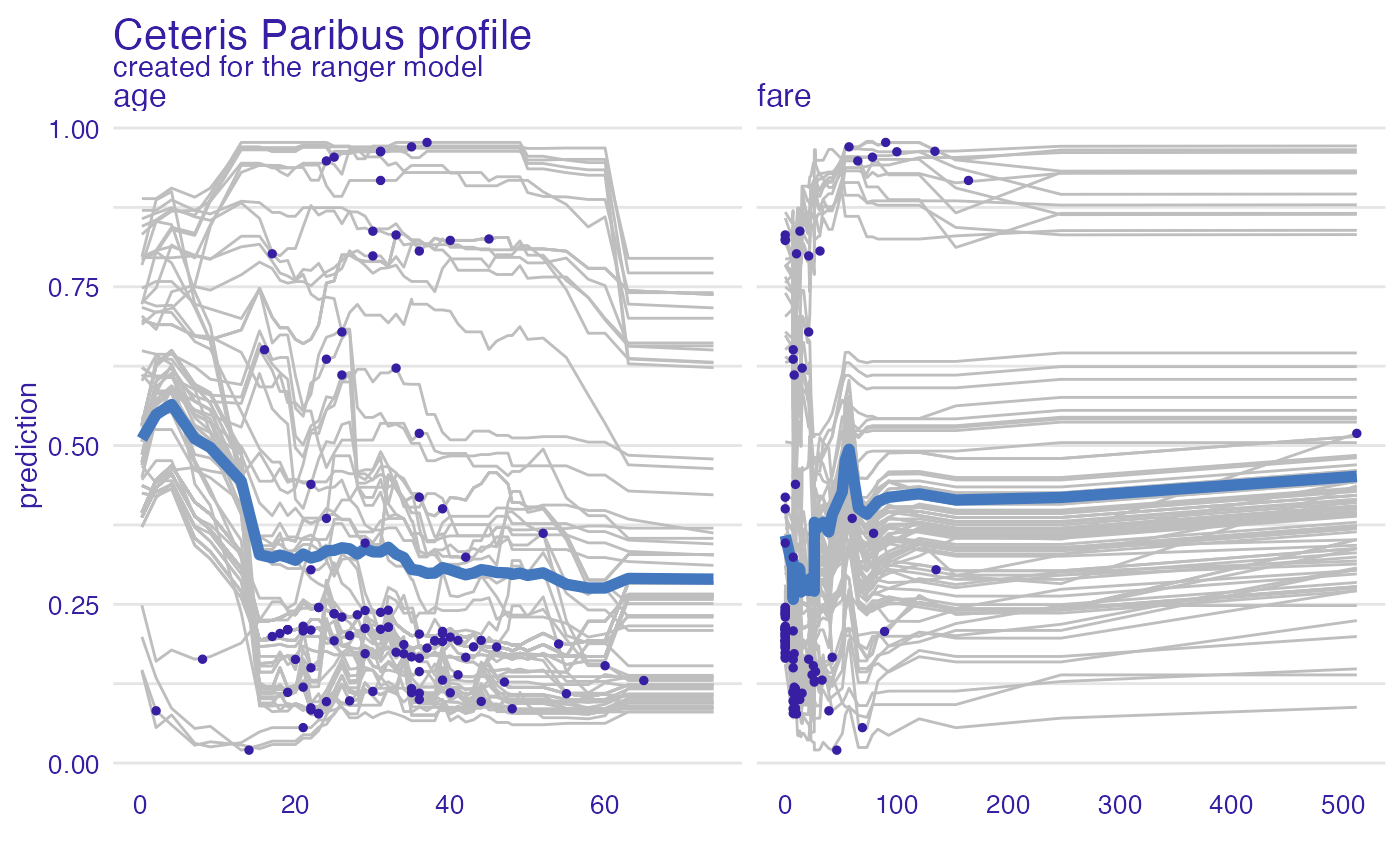vp_ra <- model_profile(explainer_ranger, type = "partial", k = 3)
plot(vp_ra)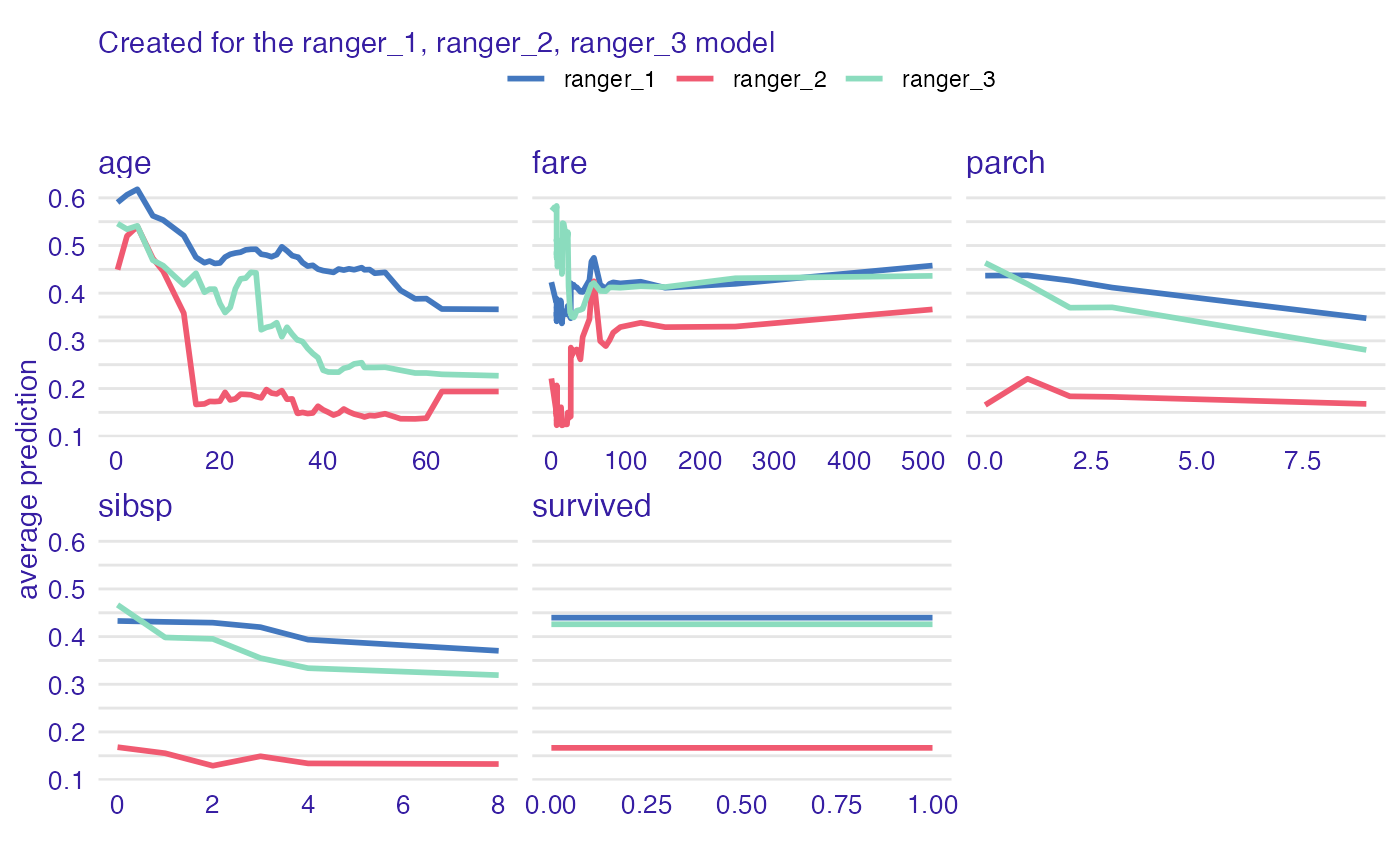plot(vp_ra, geom = "profiles")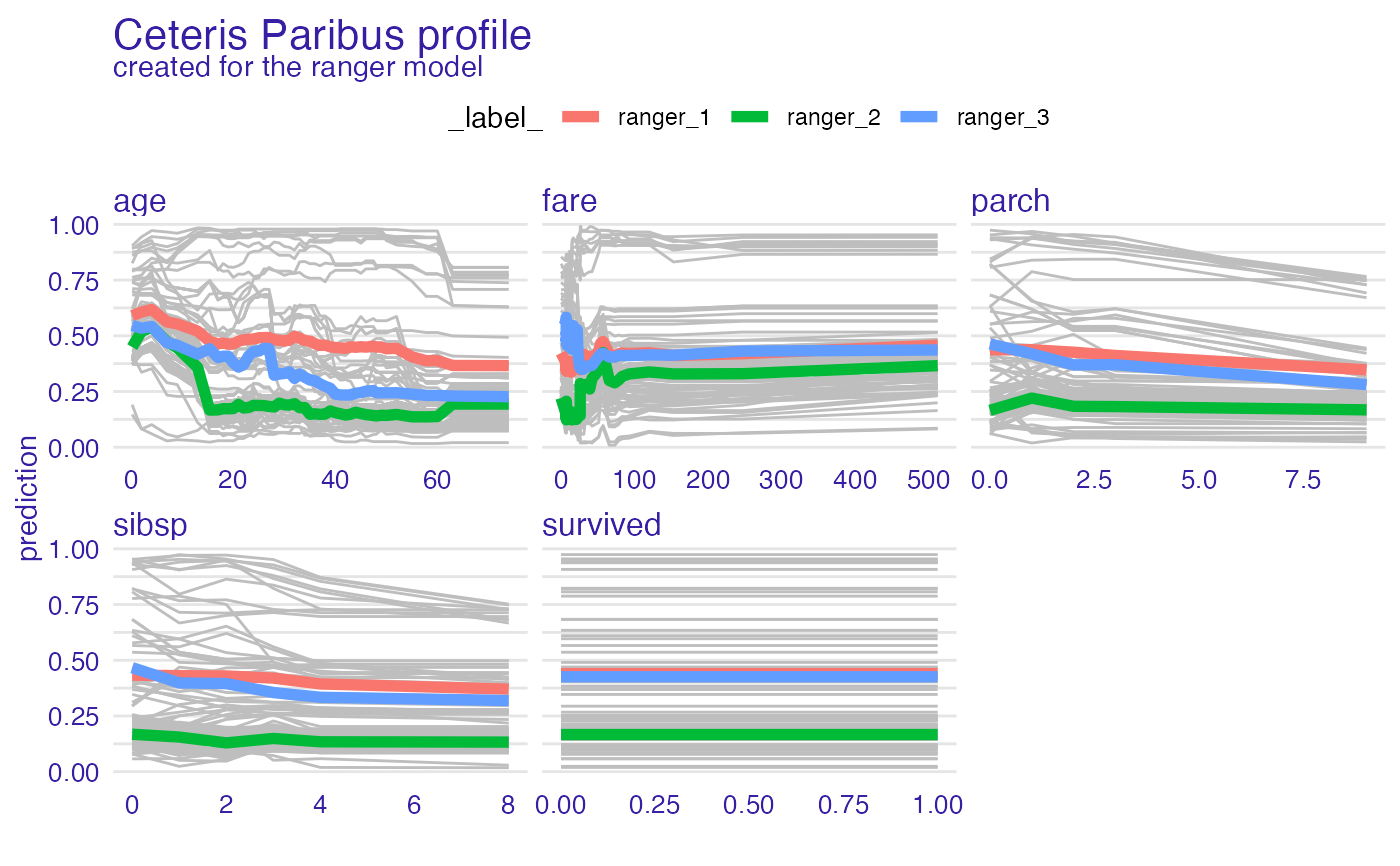plot(vp_ra, geom = "points")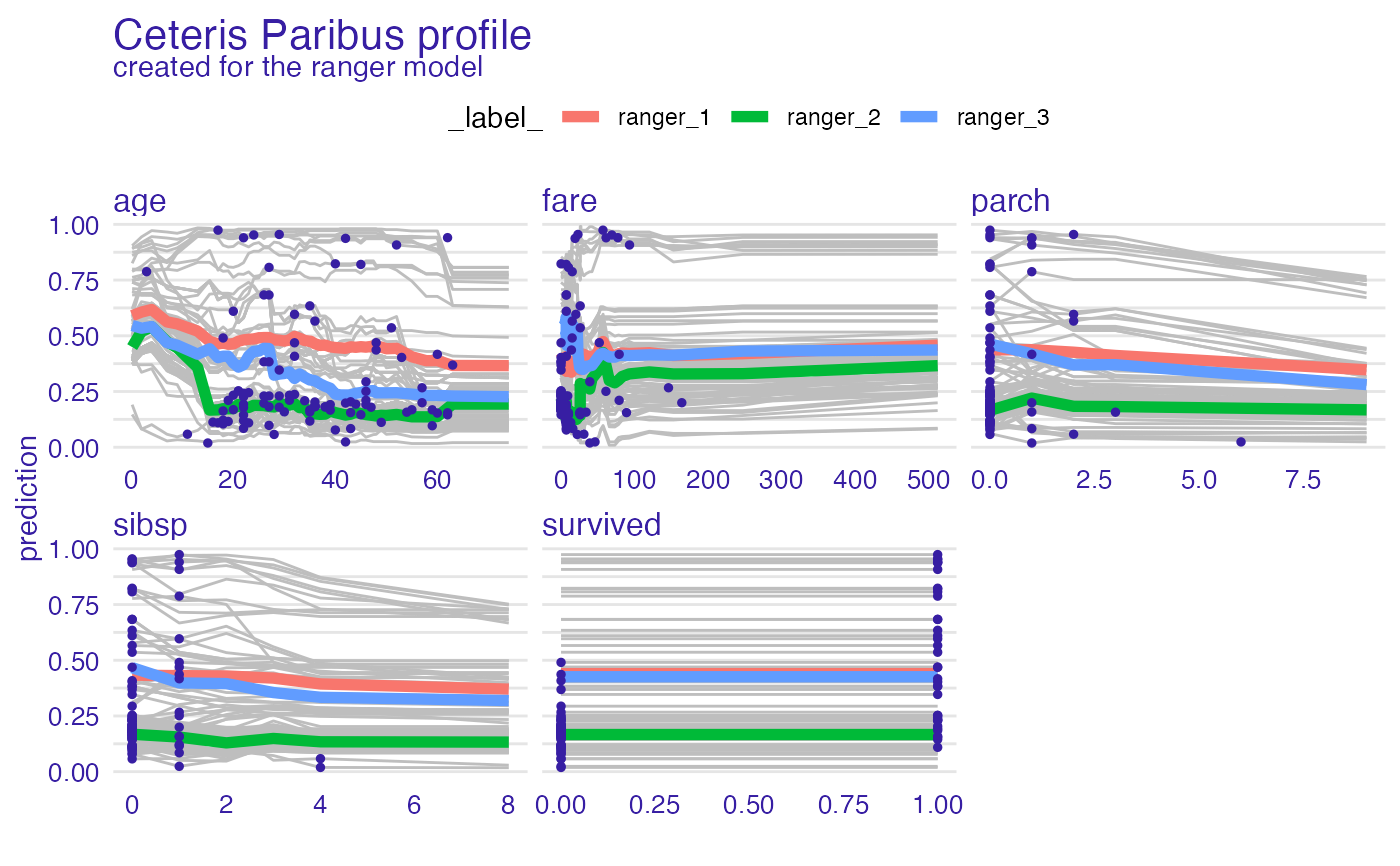vp_ra <- model_profile(explainer_ranger, type = "partial", groups = "gender")
plot(vp_ra)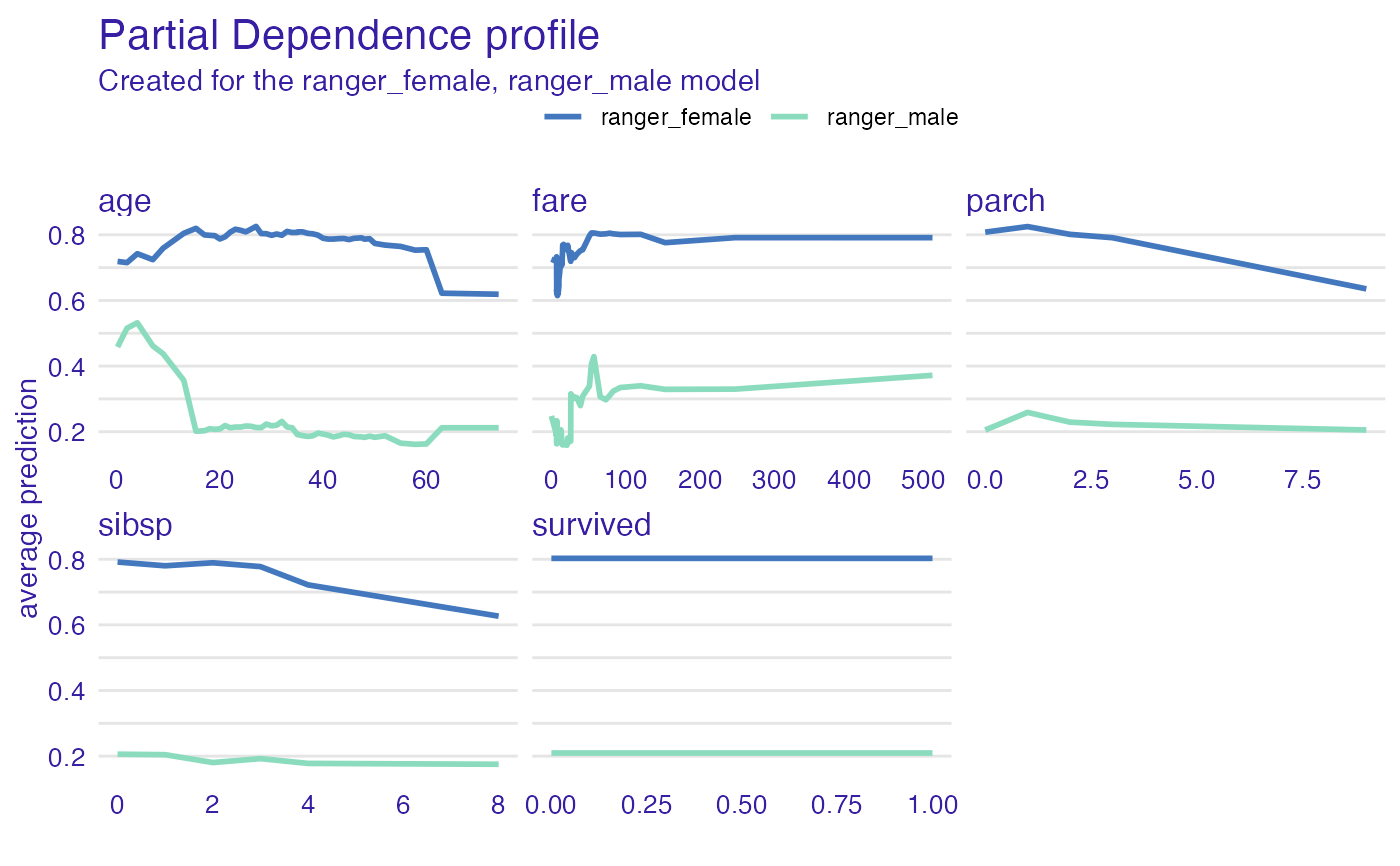plot(vp_ra, geom = "profiles")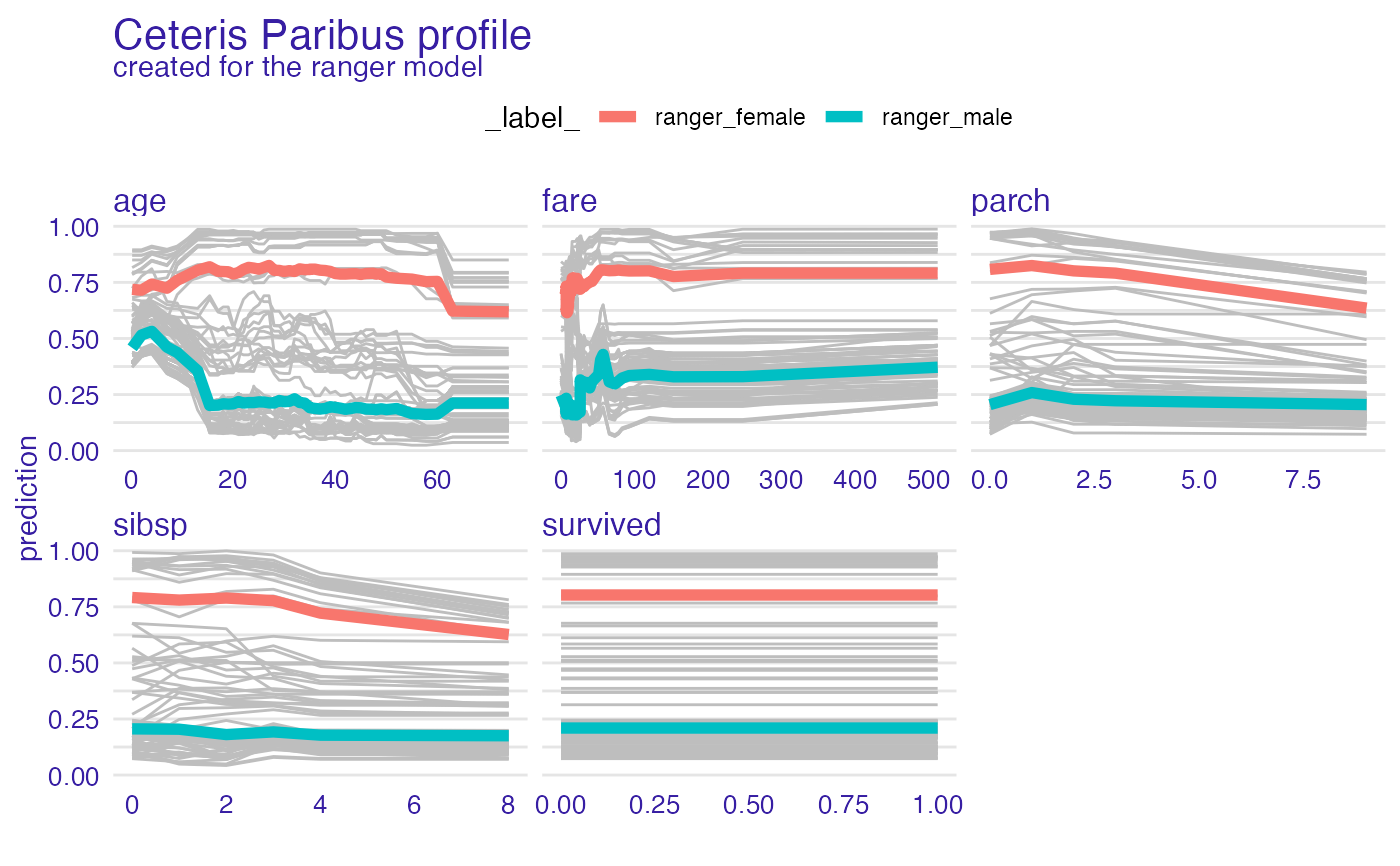plot(vp_ra, geom = "points")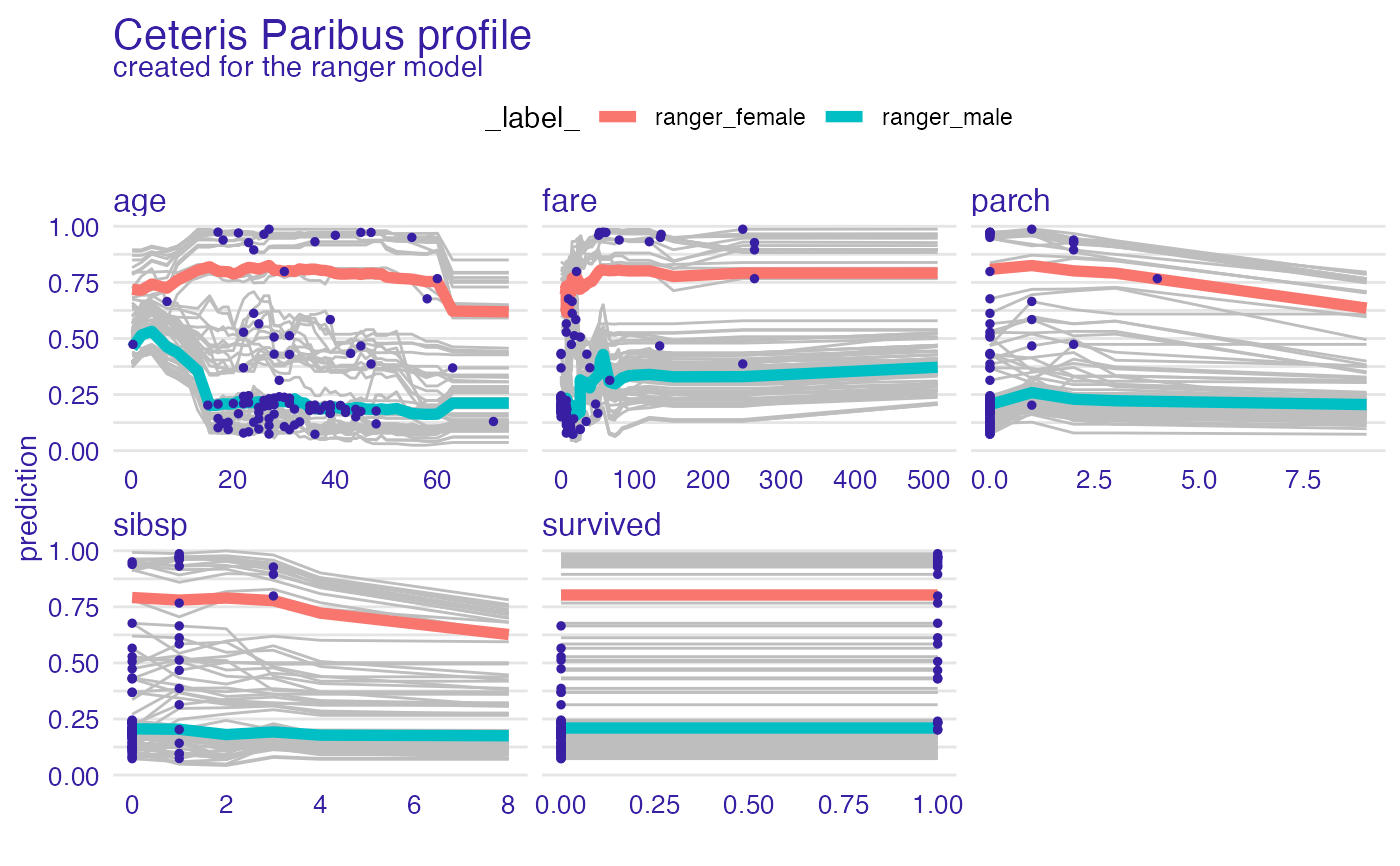vp_ra <- model_profile(explainer_ranger, type = "accumulated")
plot(vp_ra)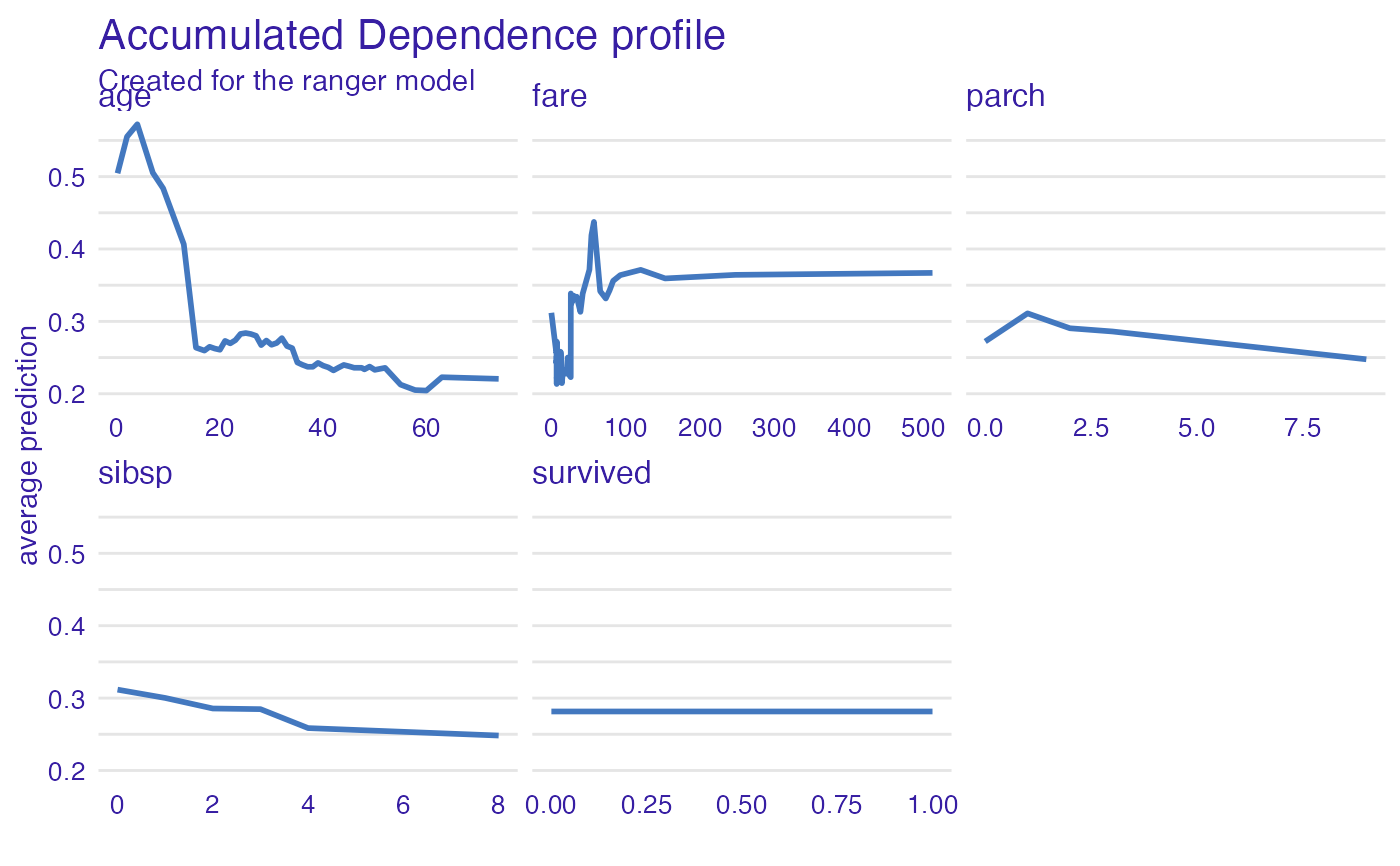plot(vp_ra, geom = "profiles")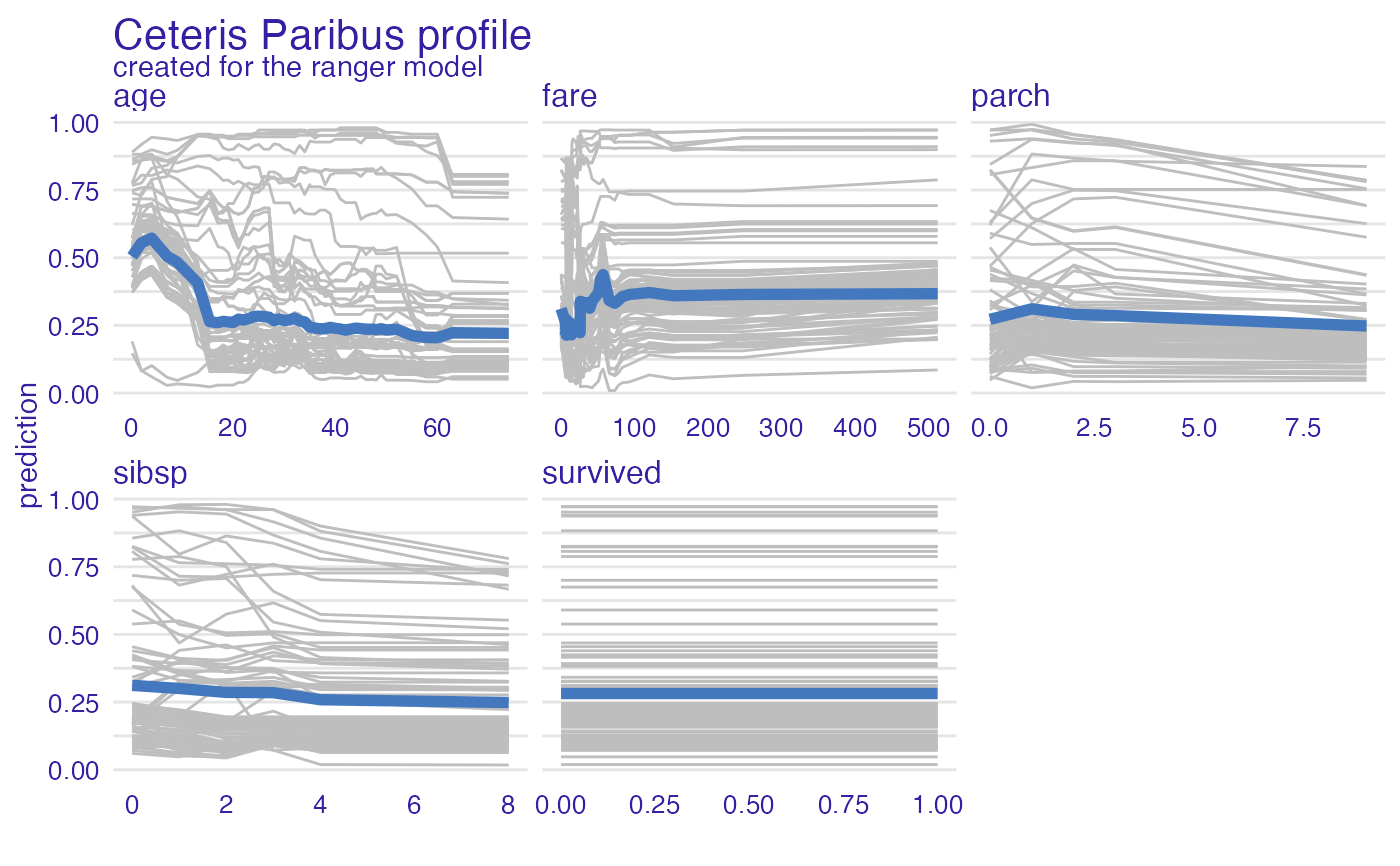plot(vp_ra, geom = "points")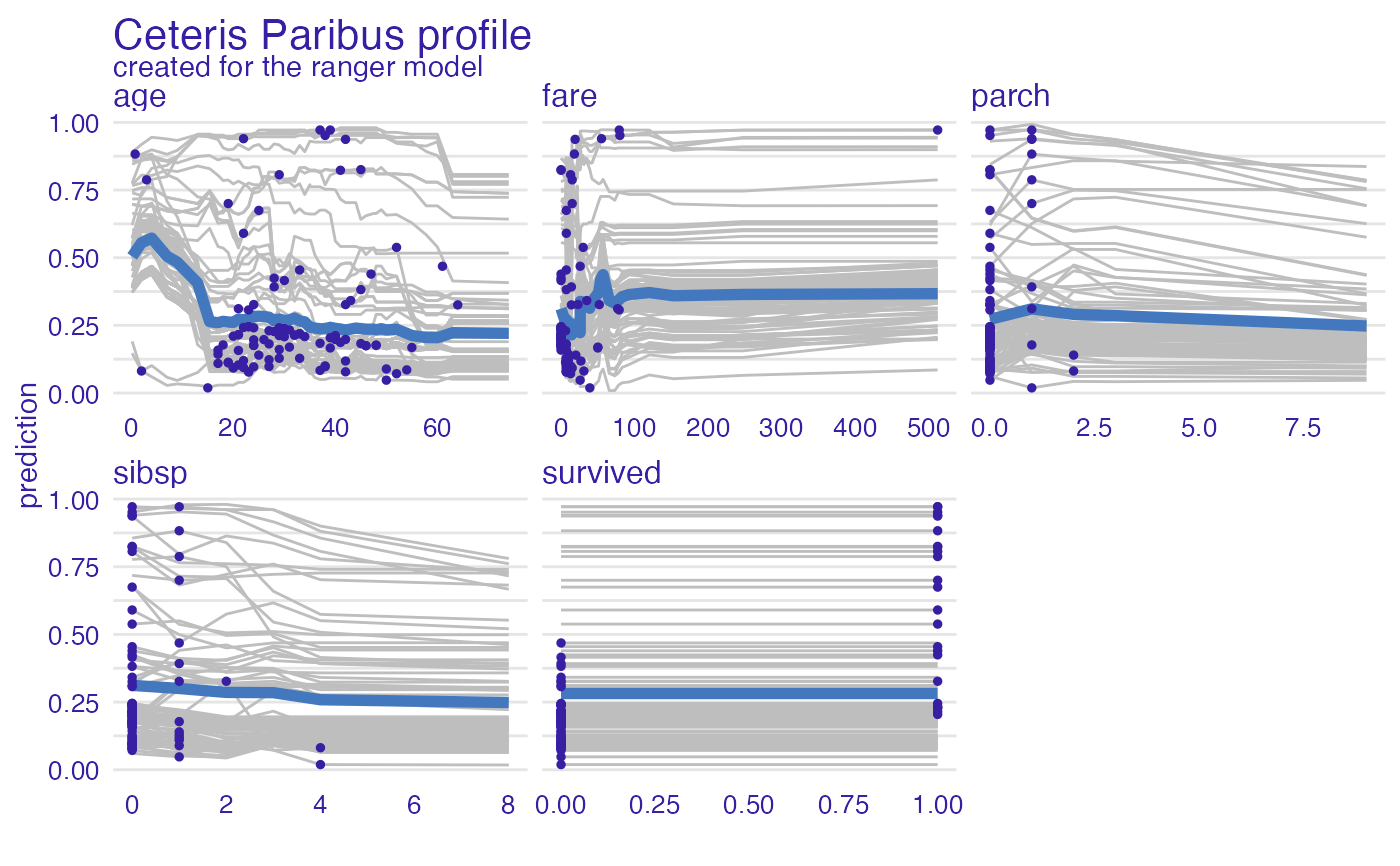# }